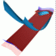Abstract and Contents
Up: 5. Examples
Next: 5.2 Quasiperiodically forced Hénon map
Previous: 5. Examples

# 5.1 The 3D-fattened Arnol'd family

The 3D-fattened Arnol'd family is defined on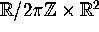by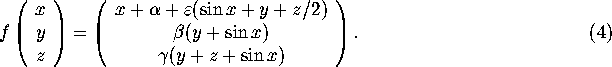Here,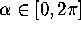,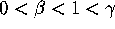and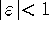. This family is introduced in [Osinga 1996, Broer et al. 1996, Broer et al. 1997] as an example of a system with a normally hyperbolic invariant circle of saddle-type. It is a three-dimensional embedding of the standard Arnol'd family of circle maps, as can be seen by setting; see [ Arnol'd 1965]. The dynamics on the normally hyperbolic invariant circle of (4) is organized as for the one-dimensional family. Forthe dynamics is periodic or quasi periodic, depending on whetheris rational or irrational. For positivethe points in parameter space where the dynamics on the circle is phase-locked form regions, the equivalents of the so-called Arnol'd tongues.

We study the 3D-fattened Arnol'd map (4) for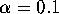,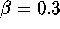,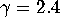, and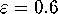. For these parameter values the invariant saddle-circle is still normally hyperbolic, and it can be computed by using the adapted graph transform of [Osinga 1996]. On the circle two saddles exist; one is attracting and the other is repelling on the circle. The situation is as sketched in Figure 1 (right). Let us consider the stable manifold of the circle. The first order approximation of this manifold is given by the data from the computations of the invariant circle.

The result of our computations is shown in Figure 10. We computed one branch of the stable manifold, starting on the linear approximation at distancefrom the circle. The invariant circle of the 3D-fattened Arnol'd family is special in the sense that it can always be parametrized by the x-coordinate if the circle is normally hyperbolic. Therefore, it is natural to choose the linear foliationwith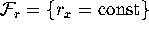. The algorithm computes intersections with 50 of these leaves with an accuracy of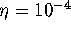. The distance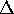between consecutive points on such intersection curves does not exceed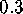. The alternatingly colored bands on the manifold have width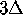. The total length of the manifold is approximately 47; note that the length of the circle is slightly more than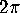.

Figure 10 also shows the two (strong) stable manifolds of the saddles on the circle. These manifolds were computed by iterating a fundamental domain on the corresponding eigenvectors. The first points are also at distancefrom the fixed points, and the iteration is done with 100 points in the fundamental domain. These curves serve as a test to show that our computations are accurate: the two curves lie in the two-dimensional manifold; see also Section 4. For high values of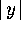and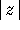, the term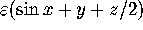in the mapping of the x-coordinate produces a strong rotational behavior. One extra iteration of the one-dimensional strong stable manifolds creates seven new windings.

Note that, if one iterates a (discretized) fundamental domain on the two-dimensional manifold, points accumulate along the one-dimensional stable manifold of the saddle that is repelling on the circle (the light blue curve in Figure 10). In other words, it is hardly possible to compute the entire surface that way.

Up: 5. Examples
Next: 5.2 Quasiperiodically forced Hénon map
Previous: 5. ExamplesAbstract and Contents

Written by: Bernd Krauskopf & Hinke Osinga
Created: May 27 1997 --- Last modified: Fri May 30 19:53:51 1997